# JP4424797B2 - 3D shape detection method - Google Patents

## Info

Publication number
JP4424797B2
JP4424797B2 JP33571599A JP33571599A JP4424797B2 JP 4424797 B2 JP4424797 B2 JP 4424797B2 JP 33571599 A JP33571599 A JP 33571599A JP 33571599 A JP33571599 A JP 33571599A JP 4424797 B2 JP4424797 B2 JP 4424797B2
Authority
JP
Japan
Prior art keywords
correspondence
image
obtained
local
degree
Prior art date
Legal status (The legal status is an assumption and is not a legal conclusion. Google has not performed a legal analysis and makes no representation as to the accuracy of the status listed.)
Expired - Fee Related
Application number
JP33571599A
Other languages
Japanese (ja)
Other versions
JP2001153633A (en
Inventor

Original Assignee

Priority date (The priority date is an assumption and is not a legal conclusion. Google has not performed a legal analysis and makes no representation as to the accuracy of the date listed.)
Filing date
Publication date
Application filed by 富士通株式会社 filed Critical 富士通株式会社
Priority to JP33571599A priority Critical patent/JP4424797B2/en
Publication of JP2001153633A publication Critical patent/JP2001153633A/en
Application granted granted Critical
Publication of JP4424797B2 publication Critical patent/JP4424797B2/en
Anticipated expiration legal-status Critical
Application status is Expired - Fee Related legal-status Critical

## Images

•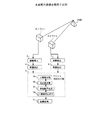•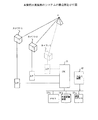•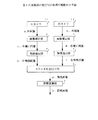•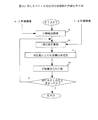•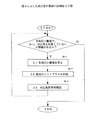•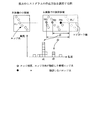•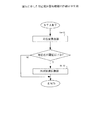•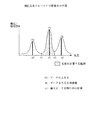•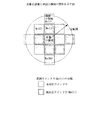•••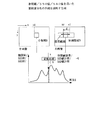•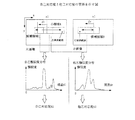•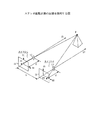## Description


BACKGROUND OF THE INVENTION
The present invention is used in a wide range of industrial fields for extracting 3D information based on stereo images, such as 3D video communication, 3D video broadcasting, 3D image generation, 3D shape measurement, 3D object recognition, and navigation for mobile robots. 3D shape detection that can stably detect the 3D position and shape of a 3D object using binocular parallax Method About.

[Prior art]
Conventionally, various methods for detecting a three-dimensional shape using binocular parallax based on two or three or more images obtained by two or three or more imaging means have been proposed. The principle of this type of three-dimensional shape detection method will be described with reference to FIG.
The same point P on the object is projected through the lenses L1 and L2 onto the imaging surfaces I1 and I2 of a pair of imaging means (hereinafter referred to as cameras) 1-1 and 1-2 such as a television camera. Here, the imaging surfaces I1 and I2 are arranged on the same plane, the focal lengths f between the corresponding lenses from each imaging surface are equal, and the optical axes of the cameras 1-1 and 1-2 are parallel to each other and b. Suppose that they are installed only apart.

Even if the pair of imaging units 1-1 and 1-2 is arbitrarily arranged, by measuring the geometric relationship between the cameras in advance, the captured image can be converted into an image obtained in the above state. It is possible to convert. Therefore, the discussion proceeds based on the above state. At this time, the point P on the object is observed at the positions of p1 (p1x, p1y) and p2 (p2x, p2y) in the captured image. Here, the positional shift on the image of the corresponding points in both images is called parallax d. Here, d = p2x−p1x.
The three-dimensional coordinates (X1, Y1, Z1) of the object point p when viewed from one camera (here, camera 1-1) are expressed as the following equations (1a) to (1c).

Z1 = f · (b / d) (1a)
X1 = p1x. (B / d) (1b)
Y1 = p1y · (b / d) (1c)

Here, Z1 is the distance from the camera 1-1, f is the focal distance of the camera, b is the distance between both cameras, d is the parallax, X1 is the three-dimensional X coordinate from the camera 1-1, and p1x is from the center of the image. The X coordinate, Y1 is the three-dimensional y coordinate from the camera 1-1, and p1y is the Y coordinate from the center of the image.
Thus, if the projection point in both images for an arbitrary point in the three-dimensional scene is determined, the coordinate value and the parallax value in the image of the projection point can be obtained, so the above formulas (1a) to (1c) A three-dimensional position can be obtained. This is the principle of three-dimensional position calculation by binocular vision. Even when three or more image pickup means are used, if any two image pickup means are considered, it is the same as described above.

As is apparent from the above principle, in order to obtain a three-dimensional position using parallax, it is necessary to obtain the corresponding projection points of the same object in both images. This is called a “correspondence search problem”.
Hereinafter, the “correspondence search problem” will be described in more detail.
Consider a stereo image obtained by a pair of imaging means. Attention is paid to a point p1 in one image (here, left image). At this time, the point p2 corresponding to p1 in the other image (here, the right image) is located on a constraint line called an epipolar line determined from the positional relationship between the two cameras.

Here, since the image conversion is performed when the optical axes of the two cameras are equivalent to each other, the epipolar line is the same scanning line (the same y coordinate), and p2 is to the left of the position where p1 is copied to the other image. Located in. Therefore, in the corresponding point search, for each position (p1) of the left image, the most similar part is scanned on the same scanning line in the right image while scanning only the search range R toward the left from the x coordinate of p1. A corresponding set can be obtained. At this time, since it is difficult to determine the degree of similarity using only points, the degree of similarity is obtained using information within a certain range including the points.
In general, since a plurality of correspondence candidates exist in the search range, it is not easy to determine a true correspondence location, and how to obtain the correspondence location correctly is the main point of three-dimensional distance measurement using binocular parallax.

The typical methods proposed so far can be broadly classified as follows.
(1) A method for obtaining the correspondence independently at each position of the image.
(2) A method for obtaining a response at each position of an image based on some support in the surrounding area or the entire image.
As a typical example of the above (1), one image is divided into small regions having a certain size, for each small region, for each pixel of one image, or characteristics of one image. The other image is scanned with respect to the point, and the similarity of the luminance distribution in the small area is evaluated by normalized correlation, the sum of absolute values of luminance differences (SAD), the sum of squares of differences of luminance values (SSD), etc. Some of them correspond to the most similar parts.

The above (2) includes the following.
(a) A method of limiting the magnitude of parallax change assuming that the distance change in the vicinity is small.
(b) The best overall response is achieved by loosely minimizing the total sum of distortion energy defined by the difference in correspondence (corresponding energy) obtained in the local area and the difference in parallax between adjacent areas. Obtaining energy minimization method.
(c) Focusing on the reliability of the feature value to be matched, the correspondence is obtained in order from the highly reliable edge point, and the correspondence information of the edge point having higher reliability already obtained for the correspondence of the edge point having lower reliability is used. What to do.

Here, (c) Method based on feature reliability (Yamaguchi, Nakayama, Shirai, Asada “Multistage Stereo Method Prioritizing Highly Reliable Response”, IEICE Transactions, Vo1, J74-D-II, No. 7, 1991) will be described.
According to this, there are the following two features when the feature point (edge point) where the brightness value changes suddenly is used as the feature point to be associated.
i) The position information of the edge point is more reliable as the luminance change (contrast) is larger, and is more unstable as the luminance information is lower.
ii) Since the number of edge points with high contrast in the image is small, the number of correspondence candidates is small and the correspondence can be easily performed.
Focusing on these features, the edges are classified into multiple groups according to the size of the contrast value in advance, and first, the correspondence of edge points that belong to the group with the highest contrast and can be easily associated is determined. Associate edge points of low contrast groups.

[Problems to be solved by the invention]
First, in the method (1) for obtaining the correspondence independently at each position of the image, the correspondence is obtained independently at each position of the image. However, if they are independently associated, if there are multiple objects with similar shapes in the target scene or there are objects that contain repeated patterns, the difference in similarity between the true corresponding part and the false corresponding part is Since it is slight, it easily corresponds to an erroneous portion due to the noise of the image and the influence of illumination. It may actually indicate the distance of the position where there is no object.
Next, the problem of a method for obtaining a response based on some support around (2) will be described.

First, in the method (a), instead of obtaining the correspondence of the target portion independently, a stable correspondence is obtained by limiting the magnitude of the parallax change with the adjacent area and reflecting the surrounding correspondence situation. ing.
However, in most cases, the target portion provided in the image is scanned in a predetermined order and processed regardless of the difficulty level of the association. If the corresponding part is mis-corresponding, the influence is transmitted to the subsequent stage, and as a result, mis-corresponding in a wide range occurs.

Next, in the method (b), there is a consistent response by considering the consistency of the entire response, not the image part, by energy minimization. Is necessary and impractical.
In the method (c), the grouping is divided according to the size of the contrast, but since the edge points belonging to the same contrast group are determined in the conventional fixed order, the repeated pattern is used. There is a problem of mis-response propagation due to order.
In addition, when an error occurs in correspondence with a high-contrast edge, the information is used for associating with an edge point having a lower contrast in the subsequent stage, and as a result, erroneous information is propagated over a wide range and the miscorrespondence increases. Furthermore, since only a small amount of edge points with high contrast are used in the initial stage of association, there is a problem that the number of edge points to vote for the parallax histogram is small, the histogram shape becomes unstable, and it becomes weak due to the repeated pattern.

The present invention has been made in consideration of the above-described circumstances, and it is possible to stably obtain the correspondence of the repeated pattern portion, which has conventionally been difficult to associate, and to obtain the correct correspondence from the entire image. Shape detection Method Is to provide.

[Means for Solving the Problems]
The present invention will be described in the case of using two imaging devices that are based on a method of acquiring three-dimensional three-dimensional information.
Information in a form having a value for each element in a two-dimensional array is defined as an image. As shown in FIG. 1, the target scene is imaged using imaging means 1-1 and 1-2 such as a pair of television cameras to obtain two images. This is called a stereo image. If the optical axes of the pair of imaging units 1-1 and 1-2 are parallel to each other and the imaging surfaces are not on the same plane, the camera external (geometric) parameters and camera internal parameters acquired by the image correction unit 3 are used. Is used to correct the image so as to be equivalent to the image obtained by the pair of imaging units 1-1 and 1-2 placed in parallel with each other, and the feature extraction unit 4 obtains a feature image for every two images. Extract.

Next, the feature image is used as an input, and the stereo correspondence unit 5 associates the two images by determining projection points of the two images of the solid 2 in the scene, and a positional shift between the two images ( Binocular parallax (hereinafter simply referred to as parallax) is obtained, and the distance conversion unit 6 determines the three-dimensional position from the camera 1-1 according to the above equations (1a) to (1c).
The projection point of the same solid 2 in the two images is obtained by searching for a similar part from the other side image for a certain part of one image. Here, if there are a plurality of solids having a similar shape in the target scene or an object including a repetitive pattern, there are a plurality of similar parts due to the similar patterns, and the correspondence becomes ambiguous. In such a case, if it is associated with the most similar part, there is a possibility that a three-dimensional position different from the actual one is calculated in correspondence with an erroneous part easily due to the influence of image noise or illumination.

Therefore, in the present invention, the uniqueness of correspondence between the two images is used as an index of stability of correspondence, and the correspondence is made in the order of high degree of correspondence stability (uniqueness) regardless of the position of the target region. This prevents the propagation of incorrect correspondence information due to the fixed scanning order. This makes it possible to obtain a correct response without being affected by other effects even in the presence of a repetitive pattern.
In addition, when finding the correspondence of parts with locally similar patterns such as repeated pattern parts and low stability, the correspondence information is obtained with the help of the disparity values of the parts with high stability that have already been found. And gradually get a stable response. In this way, since the correspondence is obtained in the optimum order in the sense that the correspondence is unique and there is no ambiguity, a stable correspondence can be obtained even in the presence of a repetitive pattern.
The uniqueness of the above correspondence is obtained as follows. In the similarity distribution obtained by scanning the candidate area with some similarity defined by the target area in one image and the corresponding candidate area in the other image, the highest similarity part is compared to the next highest part. It is assumed that the more prominent it is, the higher the uniqueness.

That is, in the present invention, as shown in FIG. 1, the stereo association unit 5 includes a small area dividing unit 5a that divides a captured scene image including the same object into small areas, and each small area of one image. On the other hand, a correspondence calculation unit 5b that evaluates the uniqueness of the correspondence of the local image within the search range of the other image and determines the correspondence, a processing order determination unit 5c that determines the processing order based on the correspondence, A small region association unit 5d that associates the small regions with each other in the processing order is provided. Then, the correspondence degree calculation unit 5b creates a similarity distribution in the search area for each small area for which correspondence is not obtained, and obtains a correspondence degree that determines the possibility of correspondence from the distribution of similarity degrees. Next, the processing order determination unit 5c arranges the components in order from the one with the best correspondence, and the small region association unit 5d sequentially obtains the correspondence from the portion where the stable correspondence is obtained.
In addition, for a portion of the similar pattern or the like for which correspondence is not obtained locally, a correspondence that is gradually stabilized can be obtained by using correspondence information of a portion in the surrounding that has already been obtained.

When using edges, all feature points in the region of interest are used regardless of contrast. When using luminance values, the luminance value distribution in the region of interest is used as is. It is possible to acquire a stable correspondence by obtaining a stable similarity distribution with a large number of features.
As a result, the part where the correspondence is clear can be found stably without being influenced by others, and the part where the correspondence is unclear due to the repeated pattern etc. is stable by using the correspondence information of the small area from which the correspondence has already been obtained. Can be obtained.
Therefore, since the correct parallax can be stably obtained over the entire image, the three-dimensional position and shape of the solid can be obtained correctly.
Also, in the above, the degree of correspondence of the small area is calculated using the degree of self-correspondence when the correspondence from one image is obtained to the same image and the degree of self-correspondence when the correspondence is obtained from one image to the other image. You may ask for it.

DETAILED DESCRIPTION OF THE INVENTION
FIG. 2 is a diagram showing a configuration example of the system according to the embodiment of the present invention. In the figure, 11 is a CPU, 12 is a display device such as a CRT or liquid crystal display, an input / output device composed of input devices such as a keyboard and a mouse, 13 is a memory composed of ROM, RAM, etc., 14 is a program, An external storage device 15 for storing data and the like is a communication interface 15 including a modem for performing data communication using a telephone line, a network card for performing data communication using a network such as a LAN, and the like.

The target scene imaged by the imaging devices (hereinafter referred to as cameras) 1-1 and 1-2 is input to the CPU 11 via the interface I / F. A program for performing the processing of the present invention is stored in the external storage device 14. The target scene input to the CPU 11 is processed by the above program to detect a three-dimensional shape, and the processing result is stored in the external storage device 14. And output from the input / output device 11. In addition, the third camera 1-3 is provided, the target scene imaged by the third camera 1-3 is input to the CPU 11, and in addition to the target scene imaged by the cameras 1-1 and 1-2, You may make it detect a solid shape using the object scene imaged with the camera 1-3.

Next, the outline of the association processing of the first embodiment of the present invention will be described with reference to FIG. In addition, although the case where the target scene is imaged using two cameras will be described here, the correspondence of each of the target scenes captured by these cameras using three or more cameras as described above, You may make it detect a solid shape using a thing with a higher correspondence degree.
In FIG. 3, the target scene is imaged by the cameras 1-1 and 1-2, and two gray images (right and left gray images) as a result are obtained. Here, the positional relationship between the pair of cameras 1-1 and 1-2 is arbitrary, but it is assumed that the geometric relationship between the two cameras is obtained in advance by some means. Here, for convenience, the camera 1-1 is called a left camera, and the camera 1-2 is called a right camera. In the following, according to this rule, the left and right prefixes are used to distinguish the outputs of the two cameras.

Next, in the image correction unit 3, the optical axes of the two cameras 1-1 and 1-2 are parallel and the imaging surfaces of the two images captured by the two cameras 1-1 and 1-2 are the same. The image correction unit 3 corrects the two gray images a and b using the geometric relationship between the cameras so as to obtain an image that can be observed when the relationship becomes a plane (reference arrangement), and the right and left corrected gray images. c and d are obtained.
In the above correction, if the rotation angles of the X, Y, and Z axes of the camera in the reference position relative to the camera at an arbitrary position are θ, φ, ξ, and the secondary distortion coefficient is κ, the image coordinates pc in the reference position (Xc, yc) is expressed by the following equations (2a) and (2b) using the coordinates po (xo, yo) of the image captured at an arbitrary position.

[Expression 1]

With this relational expression, both images captured by an arbitrarily arranged camera can be converted into an image captured by a referencely arranged camera. The feature extraction unit 4 obtains feature images e and f (edge points, edge strength, and edge direction) for each of the two images from the corrected grayscale images c and d.
The method for obtaining the edge point, edge strength, and edge direction is not mentioned here.For example, the edge point is obtained from the zero crossing point of Laplacian Gaussian applied to the grayscale image, and the edge strength is obtained from the grayscale image. It is also possible to obtain the magnitude of a vector obtained by multiply-acting Deent Gaussian and the edge direction in the direction of the vector.
The stereo associating unit 5 performs associating processing between the edge points of the two images using the feature images e and f as inputs, and obtains a parallax image g having a parallax value for each edge point position. From the parallax image g, the distance conversion unit 6 calculates the distance h of the object using the relational expressions shown in the expressions (1a) to (1c).

Next, details of the stereo correspondence processing unit 5 in FIG. 3 will be described with reference to FIG.
As shown in FIG. 4, the stereo association processing unit 5 first divides the left feature image into small regions of a certain size in the small region dividing unit 5a. At this time, the small areas may be arranged so as not to overlap each other, or the small areas may be arranged so as to overlap each other to some extent. Here, what is arranged by shifting by half the size of one side of the small area is considered.
Next, in the correspondence degree calculation unit 5b, for each small region for which correspondence is not obtained (hereinafter referred to as an unsupported small region), the correspondence between the edge points to which the correspondence belongs and the correspondence candidate points are used to determine whether the correspondence is good or bad. Find the degree. Here, the correspondence candidate point is similar to the edge to be matched with the edge attributes (edge strength and edge direction) in the edge point group in the correspondence search range on the epipolar line of the other feature image (right feature image). Refers to things. Finding the correspondence level means obtaining a parallax representing a small area (referred to as a representative parallax) and determining how stable the parallax is.

Subsequently, in the processing order determination unit 5c based on correspondence, the non-corresponding small regions are arranged in descending order of correspondence, and the higher α% is set as “corresponding processing target small region”. Here, instead of the upper α%, the upper β may be used.
Next, for each of the small areas designated as “corresponding processing target small areas”, the small area associating unit 5d checks the other feature image for each edge point belonging to the small area. Then, if there is actually an edge point at a position corresponding to the representative parallax of the small area, the edge point pair is associated. Thereby, the parallax value for each edge point is obtained. It is written in the parallax image g described above. Further, if there is an actually associated edge point, that fact is recorded.
Next, it is checked whether or not one or more corresponding points have been obtained. If one or more corresponding points are obtained, the corresponding degree calculation unit 5b returns to the surrounding non-corresponding small region (described later) to further deal with it. Repeat a series of processes from good to bad. When no corresponding point can be obtained, the process is terminated.

Next, the correspondence calculation unit 5b will be described with reference to FIG. In the correspondence level calculation unit 5b, first, it is checked whether there is a small region that is not yet supported and the correspondence level has not yet been calculated, and an uncorresponding region is obtained (5b-1).
Then, a disparity histogram is created for the unsupported region, and a corresponding similarity distribution is obtained (5b-2).
Subsequently, the correspondence calculation processing unit 5b-3 actually calculates the representative parallax and the correspondence of the small area. This operation is repeated for non-corresponding small areas for which the correspondence level is not obtained.

Here, a method of creating the above-described parallax histogram will be described with reference to FIG. Here, a small area with a left image is considered.
The following operation is performed for all edge points belonging to the small area of the left image. First, attention is paid to a certain edge point PLi. Here, the right and left corrected images are corrected so that they are the same as when captured by a camera whose optical axis is parallel and the imaging surface is located on the same plane, so the epipolar line of PLi is a horizontal line, and PLi is the right image. When projected onto, it becomes the position of PLi '.
The corresponding point of PLi is located on the same horizontal line as PLi ′ and on the left side of PLi ′. Here, assuming that the search range is R, scanning is performed from PLi ′ to the maximum R to the left, and among the obtained edge points, edge points whose edge strength and edge direction are similar to PLi and attribute values are correspondence candidates. Extract as a point. Then, with the parallax values defined by these corresponding point pairs, a vote is given for a parallax histogram with the parallax value on the horizontal axis and the frequency on the vertical axis.

In FIG. 6, PR1 to PR3 are obtained from the epipolar line of PLi, and PR2 and PR3 are corresponding candidates because the attribute values are similar, but PR1 is not a corresponding candidate because the attribute values are different. Shows the case. For the corresponding candidate points PR2 and PR3, the parallaxes are d1 and d2, respectively, as shown in the figure, and these are voted on the parallax histogram.
By performing the same operation for each edge point in the left small region, a parallax histogram is obtained as a similarity distribution as shown in FIG.

Next, the correspondence calculation processing unit 5b-3 illustrated in FIG. 5 will be described with reference to FIG. First, the correspondence level is calculated by the correspondence level calculation unit 5b-31 based on the similarity distribution shown in FIG. If the correspondence level is equal to or less than the threshold value, the peripheral parallax propagation unit 5b-32 determines the representative parallax based on the correspondence information of the surrounding small areas and recalculates the correspondence level. If the correspondence level is equal to or higher than the threshold value, the correspondence level is adopted.
Hereinafter, the correspondence calculation unit 5b-31 will be described in further detail.
Here, it is assumed that there is a similarity distribution as shown in FIG. Here, P1, P2,... Are set in descending order of the peak size of the similarity distribution, the parallax values giving them are d1, d2,..., And the valley positions at the left and right of the maximum peak P1 are v1, v2. . Here, the maximum peak value (Pm), peak ratio (Pr), kurtosis (Pk), and corresponding concentration (Pc) are defined as follows.

Pm = P1 / (size of one side of small area) (3a)
Pr = 1-P2 / P1 (3b)
Pk = m (4) / [m (2) · m (2)]-3 (3c)
Pc = (area from v1 to v2) / (number of edge points in the small area) (3d)

However, m (k) represents the k-th moment of the parallax frequency using the values from v1 to v2, where the parallax value is d, as shown in the following formulas (3e) and (3f). In equations (3e) and (3f), d is the parallax value, dv is the average of the parallax values, P (d) is the frequency at the parallax d, v1 is the minimum parallax value, and v2 is the maximum parallax value.

[Expression 2]

The degree of correspondence Sm is defined as in the following equation (3g) using Pm, Pr, Pk, Pc and an arbitrary mixing function f (•).
Sm = f (Pm, Pr, Pk, Pc) (3 g)
Here, f (•) is arbitrary, but can be defined as the following equation (3h) as an example. However, k1 to k4 are arbitrary mixing coefficients.
f (Pm, Pr, Pk, Pc)
= K1 · Pm + k2 · Pr + k3 · Pk + k4 · Pc (3h)

Next, the peripheral parallax propagation unit 5b-32 illustrated in FIG. 7 will be described. As shown in FIG. 9, Nw small areas existing in a certain range around the target small area are Wn (i) {i: j = 1 to Nw}, among which Nm small areas whose correspondence has already been obtained. The area group (area with hatching in FIG. 9) is Wm (i) {i: j = 1 to Nm}, and the representative parallax value of Wm (i) is Surrounding small area parallax dm (i) {i: j = 1 to Nm}.
Next, as shown in FIG. small Area parallax dm (i) is plotted on the horizontal axis and frequency is plotted on the vertical axis Surrounding small area parallax dm (i) The value of the Create a histogram for. The maximum peak of this histogram is Pd1, the next peak is Pd2, and the parallax that gives the maximum peak is dn.
Here, if the correspondence rate Nm / Nw is equal to or greater than the threshold and the maximum peak of the dm (i) histogram shown in FIG. 10 is sufficiently larger than the next peak, the parallax value dn that gives that peak is taken as the representative representative parallax. .

Next, as shown in FIG. 11, the left and right troughs of the first, second, and third peaks are obtained for the parallax histogram of the target small region, and the region between the first and second peaks (v11 to v12) is determined. , Second mountain (v21 to v22), third mountain (v31 to v32).
If the representative parallax dn obtained from the surrounding small area is included in any of the first mountain, the second mountain, and the third mountain, the parallax that gives the maximum value of the mountain is set as the representative parallax. Further, the correspondence level at that time is the average value of the correspondence levels of Wm (i).

As described above, in the present embodiment, the correspondence degree and the representative parallax are obtained, the processing order is determined in the order of the correspondence degree, the edge points of the two images are associated, and the parallax having the parallax value for each edge point position Since the image is obtained, it is possible to prevent propagation of incorrect correspondence information due to a fixed scanning order, which is a problem of the conventional method, and to obtain a correct correspondence. In addition, for parts that cannot be found locally, the correspondence information of the parts that have already been found in the surrounding area is used to gradually obtain stable correspondence. But you can get a stable response.

In the first embodiment described above, the similarity is obtained by using an edge. As the similarity, the normalized correlation value of luminance, the absolute value sum of luminance differences (SAD), the square sum of the luminance value differences (SAD) Any value of (SSD) can also be used.
The second embodiment of the present invention using the normalized correlation value of luminance, the sum of absolute values of luminance differences (SAD), and the square sum of differences of luminance values (SSD) will be described below.
The processing of this embodiment is basically the same as the processing of the first embodiment described with reference to FIGS. 2 to 11, but the similarity distribution shown in FIG. The sum of absolute values of luminance differences (SAD) or the sum of squares of differences of luminance values (SSD) is used.

That is, as shown in FIG. 12, the normalized correlation value of the luminance and the luminance calculated from the small region A to be associated with one image with the small region B having the same shape provided in the search region of the other image When any one of the sum of absolute values of differences (SAD) and the sum of squares of differences of luminance values (SSD) is V, (C−V) is used as a similarity, and a small region in the search region is scanned. While creating the similarity distribution. C is an arbitrary constant for preventing the similarity from being a negative value. The other processes are the same as those in the first embodiment, and the correspondence order is calculated based on the similarity, the processing order is determined, and the association is performed.

The normalized correlation value, the absolute value sum of luminance differences (SAD), and the square sum of the luminance value differences (SSD) will be described below.
(1) Normalized correlation
The luminance value at each position (i, j) in the small area of the left image is q L (I, j), the luminance value at each position (i, j) in the small area B of the right image is q R The normalized correlation value is expressed by the following equations (4a) to (4c) where (i, j) and the size of one side of the small region are w.

[Equation 3]

(2) Absolute sum of luminance values (SAD)
When the same symbols as in the above (1) are used, the absolute value sum E2 of the luminance difference is expressed by the following equation (5a).

[Expression 4]

(3) Sum of squares of luminance difference (SSD)
When the same symbols as in the above (1) are used, the square sum E3 of the luminance difference is expressed by the following equation (6a).

[Equation 5]

In the above embodiment, when the correspondence level calculation unit 5b-31 shown in FIG. 7 calculates the correspondence level, the other degree of correspondence when the correspondence from one image to the other image is obtained is used. In addition to the degree of self-correspondence, the degree of correspondence can be obtained from both by using the degree of self-correspondence when the correspondence is obtained from one image to the same image.
The third embodiment of the present invention using the above self-correspondence will be described below.
The processing of this embodiment is basically the same as the processing of the first embodiment described with reference to FIGS. 2 to 11, but as described above, the self-correspondence S2 is set in addition to the other-self correspondence S1. Introduce.
Self-response S2 is FIG. As shown in FIG. 5, the calculation is similarly performed from the similarity histogram determined by the small area A in the left image and the candidate area C in the left search range of the same left image. FIG. The left search range shown in FIG. 5 has the same width as the search range of the right image on the left and right sides of the target small area A. Also, the self-correspondence degree S1 is FIG. As shown in FIG. 6, the calculation is performed by the method defined in the above embodiment from the similarity histogram defined by the small area A in the left image and the candidate area B in the right search range of the right image.

The calculation of the degree of correspondence of the target small area A in the degree-of-correspondence calculation unit 5b-31 is calculated as a value using the mixed function of the two correspondence degrees S1, S2 to f2 (S1, S2). Here, f (•) is arbitrary, but for example, the following can be considered.
f2 (S1, S2) = C1 · S1 + C2 · S2
Here, C1 and C2 are arbitrary mixing coefficients.
In the present embodiment, using the correspondence degree calculated as described above, the association processing is performed by the method described in the first embodiment, and the same processing as in the first embodiment is performed thereafter. A parallax image is obtained.

【The invention's effect】
As described above, the following effects can be obtained in the present invention.
(1) The correspondence between the two images is performed in the order in which the correct correspondence is obtained based on the uniqueness of the correspondence regardless of the position of the image. Propagation of correspondence information can be prevented. Furthermore, for similar patterns, etc., where the stability is low and difficult to associate, the repetitive pattern, which was difficult to associate in the past, is determined by referring to the corresponding disparity values with high stability in the surrounding area. It is possible to stably obtain the correspondence of the parts, and to obtain the correct correspondence for the entire image.
(2) As feature quantities to be associated, when using edges, all edge points in the region of interest are used regardless of contrast, and when using luminance values, the luminance value distribution in the region of interest is used as it is. Using a sufficient number of features, a stable similarity distribution shape can be obtained.
Thereby, there is no hindrance to the formation of a remarkable similarity peak due to a small amount of false portions that may occur when some features such as high-contrast edge points are used, and correct correspondence can be obtained from the entire image.
[Brief description of the drawings]
FIG. 1 is a diagram illustrating the principle of the present invention.
FIG. 2 is a diagram illustrating a configuration example of a system according to an embodiment of this invention.
FIG. 3 is a diagram illustrating an outline of association processing according to the first embodiment;
4 is a diagram illustrating details of a stereo association processing unit illustrated in FIG. 3; FIG.
FIG. 5 is a diagram illustrating details of a correspondence calculation unit illustrated in FIG. 4;
FIG. 6 is a diagram illustrating a method of creating a parallax histogram.
7 is a diagram showing details of a correspondence degree calculation processing unit shown in FIG. 5;
FIG. 8 is a diagram illustrating a relationship between a similarity distribution and a peak.
FIG. 9 is a diagram illustrating a relationship between a target small area and a surrounding small area.
FIG. 10 is a diagram illustrating a parallax histogram of surrounding small regions.
FIG. 11 is a diagram showing a relationship between a parallax histogram of a target small region and a mountain;
FIG. 12 is a diagram illustrating creation of a similarity distribution using correlation values / SSD values / SAD values.
FIG. 13 is a diagram showing a relationship between a self-correspondence level and another self-correspondence level.
FIG. 14 is a diagram illustrating the principle of stereo distance measurement.
[Explanation of symbols]
1-1 to 1-3 Imaging means (camera)
2 solid
3 Image correction unit
4 Feature extraction unit
5 Stereo mapping part
5a Small area dividing means
5b Correspondence level calculation part
5c Processing order determination unit
5d Small region association part
6 Distance converter

## Claims (4)

1. For a stereo image obtained by photographing a scene image including the same object by two or more imaging means, the uniqueness of the correspondence of the local image within the search range of the other image is evaluated for each local region of one image. defining a degree of correspondence, based on the correspondence degree, a method of Ru associates the local area of each local region and the other image of one image in the sequence corresponding is easily obtained,
For a local region whose correspondence is not uniquely determined, in addition to the correspondence information on the local region, correspondence information on the local region that has already been found to be supported in the surrounding local region within a certain range from the local region. A three-dimensional shape detection method characterized in that a unique correspondence is obtained by using and a three-dimensional shape is detected based on the correspondence.
2. Dealing with the possibility information of a local area where the correspondence is not uniquely determined, and the possibility information of the local area that is already in the fixed range from the local area,
Determine the only response calculated from the combination of each response possibility
The three-dimensional shape detection method according to claim 1.
3. For a stereo image obtained by photographing a scene image including the same object by two or more imaging means , the uniqueness of the correspondence of the local image within the search range of the other image is evaluated for each local region of one image. It is a method of determining the correspondence and associating each local castle of one image with the local region of the other image in the order in which correspondence is easily obtained based on the correspondence.
Use the degree of correspondence defined using the degree of self-correspondence when seeking correspondence from one image to the same image in addition to the degree of other self-correspondence when seeking correspondence from one image to the other image <br / > A solid shape detection method characterized by that.
4. The solid shape detection method according to claim 1 and the solid shape detection method according to claim 3 are combined.
A solid shape detection method characterized by the above.
JP33571599A 1999-11-26 1999-11-26 3D shape detection method Expired - Fee Related JP4424797B2 (en)

## Priority Applications (1)

Application Number Priority Date Filing Date Title
JP33571599A JP4424797B2 (en) 1999-11-26 1999-11-26 3D shape detection method

## Applications Claiming Priority (1)

Application Number Priority Date Filing Date Title
JP33571599A JP4424797B2 (en) 1999-11-26 1999-11-26 3D shape detection method

## Publications (2)

Publication Number Publication Date
JP2001153633A JP2001153633A (en) 2001-06-08
JP4424797B2 true JP4424797B2 (en) 2010-03-03

# Family

## Family Applications (1)

Application Number Title Priority Date Filing Date
JP33571599A Expired - Fee Related JP4424797B2 (en) 1999-11-26 1999-11-26 3D shape detection method

## Country Status (1)

JP (1) JP4424797B2 (en)

## Families Citing this family (7)

* Cited by examiner, † Cited by third party
Publication number Priority date Publication date Assignee Title
JP4075892B2 (en) * 2002-10-22 2008-04-16 トヨタ自動車株式会社 Image processing method and image processing apparatus
JP2014132219A (en) * 2011-04-20 2014-07-17 Sanyo Electric Co Ltd Information acquisition device and object detector
JP5856888B2 (en) * 2012-03-27 2016-02-10 グローリー株式会社 Corresponding point search method, corresponding point search device, and corresponding point search program
JP6209825B2 (en) * 2013-02-15 2017-10-11 株式会社リコー Parallax detection device and parallax detection method
JP6342128B2 (en) 2013-08-23 2018-06-13 キヤノンメディカルシステムズ株式会社 Image processing apparatus, method, program, and stereoscopic image display apparatus
JP6565188B2 (en) 2014-02-28 2019-08-28 株式会社リコー Parallax value deriving apparatus, device control system, moving body, robot, parallax value deriving method, and program
JP6332703B2 (en) * 2016-02-29 2018-05-30 セコム株式会社 Space recognition device

## Also Published As

Publication number Publication date
JP2001153633A (en) 2001-06-08

## Similar Documents

Publication Publication Date Title
Coughlan et al. Manhattan world: Orientation and outlier detection by bayesian inference
Hall-Holt et al. Stripe boundary codes for real-time structured-light range scanning of moving objects
Yuan et al. Detecting motion regions in the presence of a strong parallax from a moving camera by multiview geometric constraints
US5383013A (en) Stereoscopic computer vision system
Brown et al. Advances in computational stereo
US5432712A (en) Machine vision stereo matching
US6754370B1 (en) Real-time structured light range scanning of moving scenes
US8009897B2 (en) Method and apparatus for image matching
AU2010260256B2 (en) Piecewise planar reconstruction of three-dimensional scenes
US5719954A (en) Stereo matching method and disparity measuring method
US20050286758A1 (en) Color segmentation-based stereo 3D reconstruction system and process employing overlapping images of a scene captured from viewpoints forming either a line or a grid
US8325220B2 (en) Stereoscopic image display method and apparatus, method for generating 3D image data from a 2D image data input and an apparatus for generating 3D image data from a 2D image data input
US9234749B2 (en) Enhanced object reconstruction
JP2010502945A (en) Method for determining depth map from image, apparatus for determining depth map
DE10080012B4 (en) Three-dimensional method of detecting objects and system for picking up an object from a container using the method
US20030035098A1 (en) Pose estimation method and apparatus
US6856314B2 (en) Method and system for 3D reconstruction of multiple views with altering search path and occlusion modeling
US5930378A (en) Dynamic image processing apparatus and method
US7031512B2 (en) Method and system for 3D smoothing within the bound of error regions of matching curves
JP2004234423A (en) Stereoscopic image processing method, stereoscopic image processor and stereoscopic image processing program
US8867790B2 (en) Object detection device, object detection method, and program
Rufli et al. Automatic detection of checkerboards on blurred and distorted images
EP1658579B1 (en) Method for for classification and spatial localization of bounded 3d-objects
EP0762326B1 (en) Object detecting apparatus in which the position of a planar object is estimated by using Hough transform
US20100182406A1 (en) System and method for three-dimensional object reconstruction from two-dimensional images

## Legal Events

Date Code Title Description
A621 Written request for application examination

Free format text: JAPANESE INTERMEDIATE CODE: A621

Effective date: 20060828

A977 Report on retrieval

Free format text: JAPANESE INTERMEDIATE CODE: A971007

Effective date: 20080709

A131 Notification of reasons for refusal

Free format text: JAPANESE INTERMEDIATE CODE: A131

Effective date: 20080715

A521 Written amendment

Free format text: JAPANESE INTERMEDIATE CODE: A523

Effective date: 20080916

A131 Notification of reasons for refusal

Free format text: JAPANESE INTERMEDIATE CODE: A131

Effective date: 20090929

A521 Written amendment

Free format text: JAPANESE INTERMEDIATE CODE: A523

Effective date: 20091113

TRDD Decision of grant or rejection written
A01 Written decision to grant a patent or to grant a registration (utility model)

Free format text: JAPANESE INTERMEDIATE CODE: A01

Effective date: 20091208

A01 Written decision to grant a patent or to grant a registration (utility model)

Free format text: JAPANESE INTERMEDIATE CODE: A01

A61 First payment of annual fees (during grant procedure)

Free format text: JAPANESE INTERMEDIATE CODE: A61

Effective date: 20091208

R150 Certificate of patent or registration of utility model

Free format text: JAPANESE INTERMEDIATE CODE: R150

FPAY Renewal fee payment (event date is renewal date of database)

Free format text: PAYMENT UNTIL: 20121218

Year of fee payment: 3

FPAY Renewal fee payment (event date is renewal date of database)

Free format text: PAYMENT UNTIL: 20121218

Year of fee payment: 3

FPAY Renewal fee payment (event date is renewal date of database)

Free format text: PAYMENT UNTIL: 20131218

Year of fee payment: 4

LAPS Cancellation because of no payment of annual fees